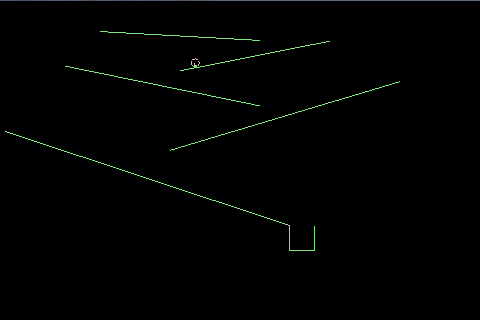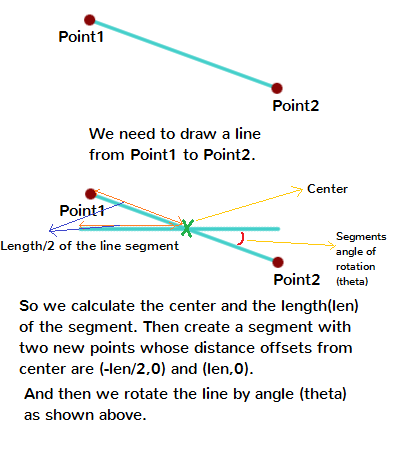# Edge Shape in Box2d

We have been working on a new 2d libgdx platformer game for sometime now and it is coming along nicely. While working on it we found the need to add an edge shape rather a rectangle for our platforms. And we found out edge shapes are quite easy to add in box2d.

At the bottom of the post we have attached the source code for this example. Here  is a screenshot.Box2D Edge Shape

http://noamchen.com/nature/north-america/ Making Edge Shape in Box2D:

For making the edge shape first we need to pass the position of the body (which would be center of the line segment in this case). After the body is create we need to attach a fixture to it whose shape would be a edge shape. In edge shape we get the option to set values for x1,y1 and x2,y2 (the ends of the line segment). As we have set the position of body at the center of line segment, we will calculate and set the offset of these two points from center as values for x1,y1 and x2,y2.

Here is a snippet on creating an edge shape.

```
public static float WORLD_TO_BOX=0.01f;
public static float BOX_TO_WORLD=100f;

static float ConvertToBox(float x){
return x*WORLD_TO_BOX;
}

static float ConvertToWorld(float x){
return x*BOX_TO_WORLD;
}

public Body CreateEdgeBody(World world,BodyType bodyType,
float v1x, // X1 WORLD COORDINATE
float v1y, // Y1 WORLD COORDINATE
float v2x, // X2 WORLD COORDINATE
float v2y  // Y2 WORLD COORDINATE
){
BodyDef bodyDef = new BodyDef();
bodyDef.type = bodyType;

//CALCULATE CENTER OF LINE SEGMENT
float posx=(v1x+v2x)/2f;
float posy=(v1y+v2y)/2f;

//CALCULATE LENGTH OF LINE SEGMENT
float len=(float) Math.sqrt((v1x-v2x)*(v1x-v2x)+(v1y-v2y)*(v1y-v2y));

//CONVERT CENTER TO BOX COORDINATES
float bx=ConvertToBox(posx);
float by=ConvertToBox(posy);
bodyDef.position.set(bx,by);
bodyDef.angle=0;

Body body = world.createBody(bodyDef);

MakeEdgeShape(body,len,bodyType,1,0,1);

//CALCULATE ANGLE OF THE LINE SEGMENT
body.setTransform(bx, by, MathUtils.atan2(v2y-v1y, v2x-v1x));

return body;
}

void MakeEdgeShape(Body body,float len,BodyDef.BodyType bodyType,
float density,float restitution,float friction){
FixtureDef fixtureDef=new FixtureDef();
fixtureDef.density=density;
fixtureDef.restitution=restitution;
fixtureDef.friction=friction;

EdgeShape es=new EdgeShape();
//SET LENGTH IN BOX COORDINATES
float boxLen=ConvertToBox(len);
//SETTING THE POINTS AS OFFSET DISTANCE FROM CENTER
es.set(-boxLen/2f,0,boxLen/2f,0);
fixtureDef.shape=es;

body.createFixture(fixtureDef);
fixtureDef.shape.dispose();
}

```

In the above snippet we are first converting the world coordinates to box as we have set a ratio of box to world to 1:100. So we scale down while calculating box values and vice versa to calculate them back to world.

After we have determined the value of center of line segment we calculate is length and angle. After that we create edge shape as a horizontal line segment with half length as its offsets in left and right direction and then rotate the body by the angle calculated above. You can calculate the offset points by just subtracting end points from the center but in that case the angle of body would be considered 0 and we needed the angle of rotation to rotate the texture attached to the line segment. So we went along with the above method.

Here is an image to describe what we did above.Attached is the source code below of the code files only and also full libgdx eclipse project workspace.

Code Files only

Full Project

1.nfsking2000 says: